网站首页 > 文章中心> 数码 > 有趣的 Scala 语言：简洁的 Scala 语法 > 正文

# 二、变量和函数

// 定义函数

def square(x: Int): Int = x * x

// 如果不是递归函数，函数返回类型可省略

def sum_of_square(x: Int, y: Int) = square(x) + square(y)

sum_of_square(2, 3)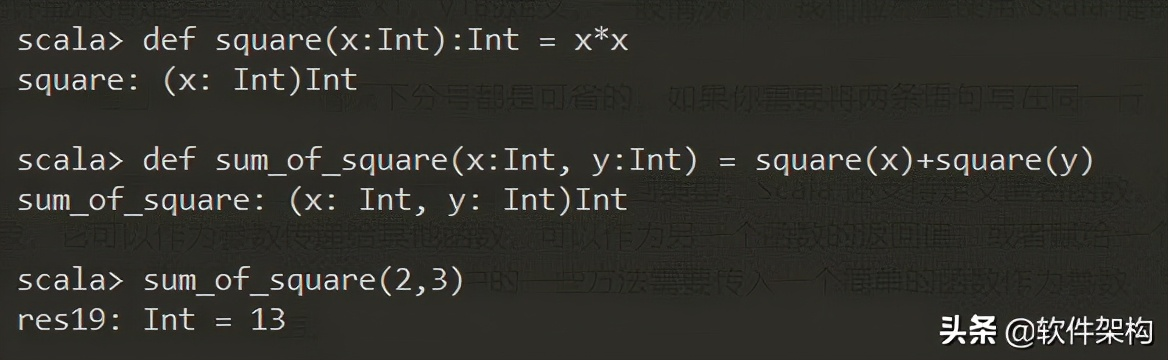// 定义匿名函数

val cube = (x: Int) => x * x *x

cube(3)

// 使用匿名函数，返回列表中的正数

List(-2, -1, 0, 1, 2, 3).filter(x => x > 0)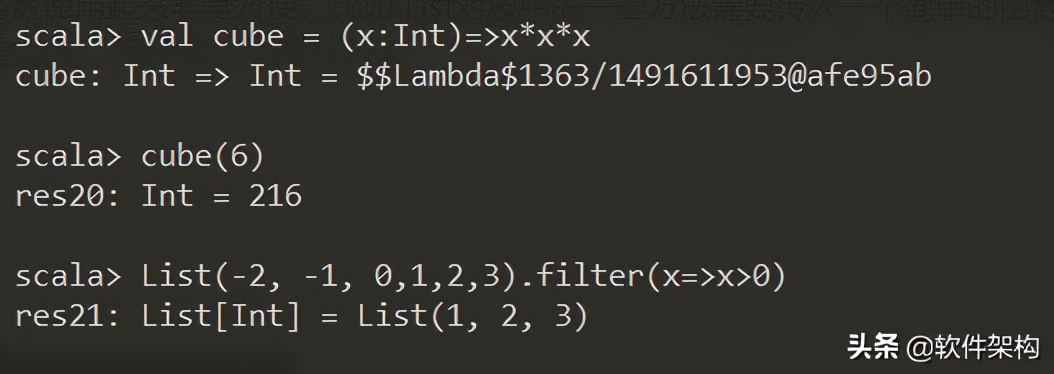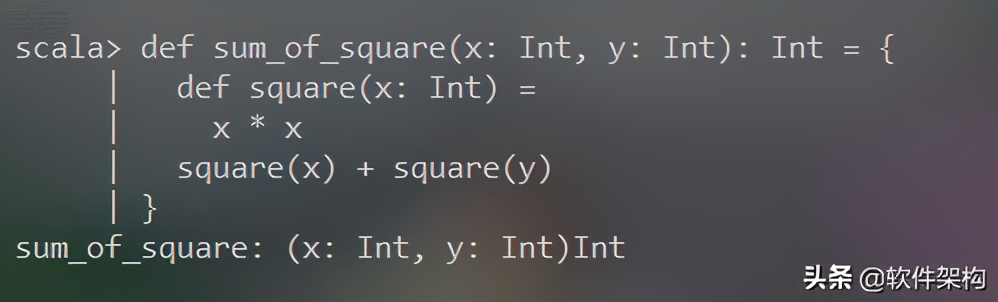# 三、流程控制语句

def abs(n: Int): Int = if (n > 0) n else -n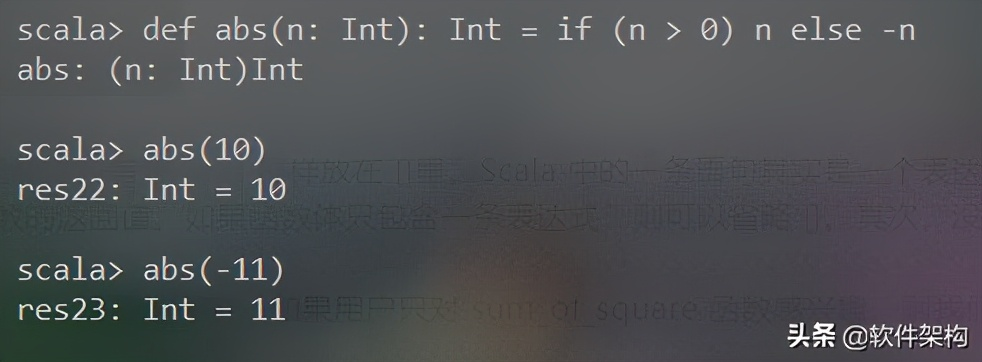def sum(xs: List[Int]) = {

var total = 0

var index = 0

while (index < xs.size) {

total += xs(index)

index += 1

}

total

}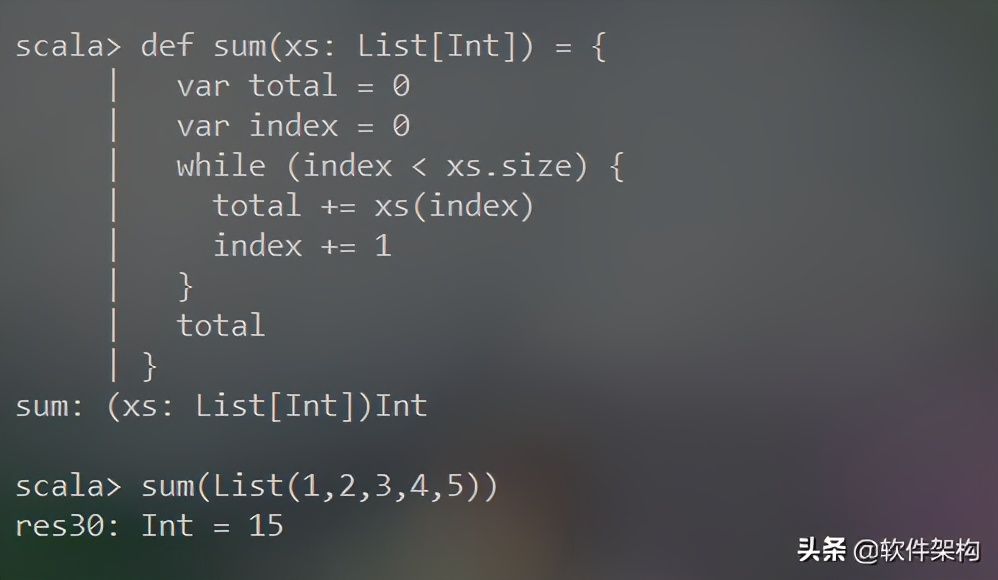//xs.tail 返回除头元素外的剩余元素组成的列表

def sum1(xs: List[Int]): Int = if (xs.isEmpty) 0 else xs.head + sum1(xs.tail)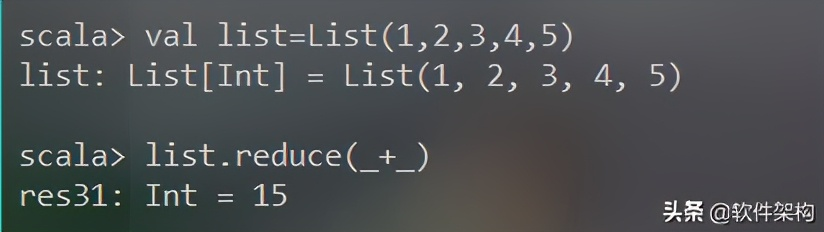list map (_.toString) reduce((x,y)=>s"f(\$x,\$y)")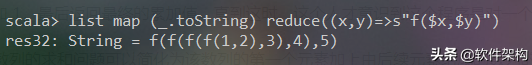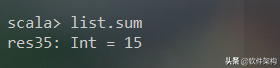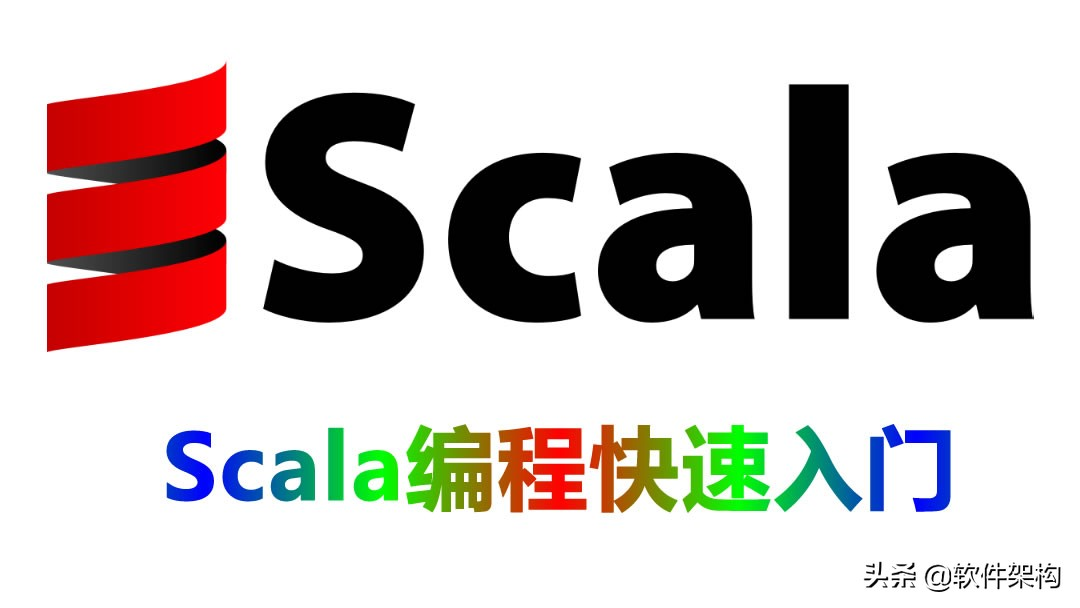# 四、如何运行 Scala 程序？

Hello.scala 代码：

println("Hello Rickie!")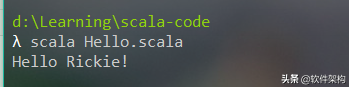object HelloRickie {

def main(args: Array[String]): Unit = {

println("Hello Rickie!")

}

}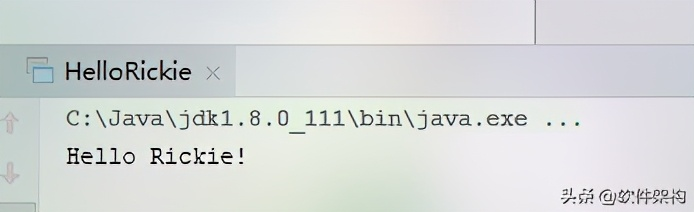Scala 还提供了一个更简便的方式，直接继承另一个对象 App，无需定义 main方法，编译即可运行。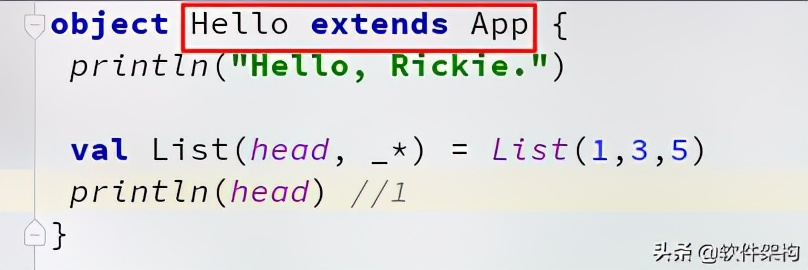# 五、结束语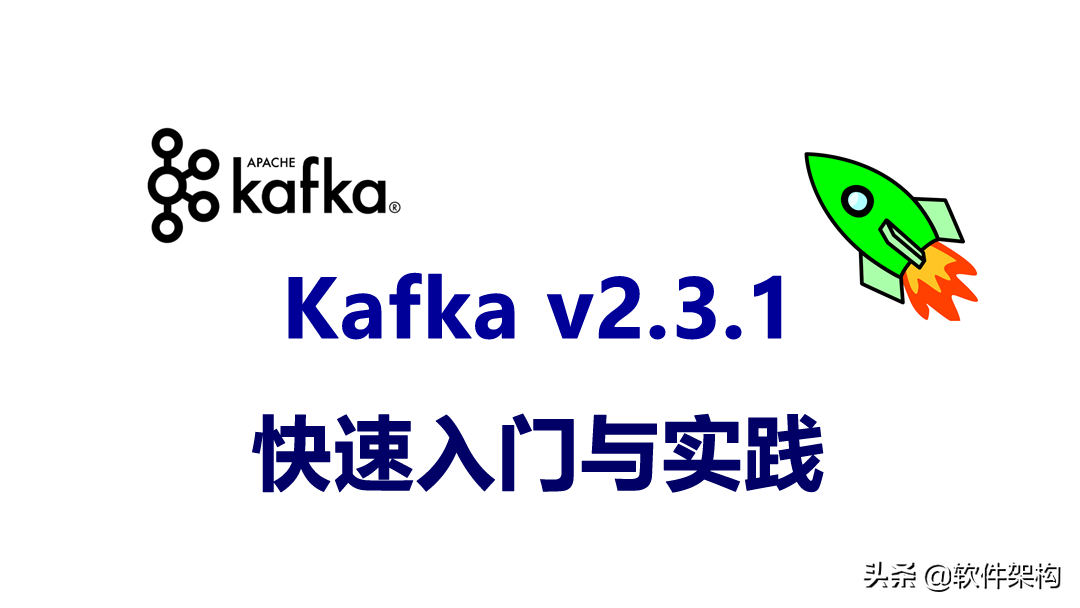9:00-22:00